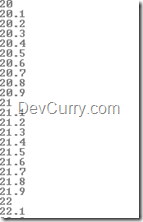# Generate Sequence of Float Numbers within a Range using LINQ

 Tweet

Sometime back, I had written a post to Generate Odd Numbers within a Range using LINQ. Here’s a small modification to use LINQ to generate float numbers within a Range say - 20 to 40.

C#

`static void Main(string[] args){    var rng = Enumerable.Range(200, 200).Select(x => x / 10f);    foreach (float n in rng)    {        Console.WriteLine(n);    }    Console.ReadLine();}`

VB.NET (Converted Code)

`Sub Main()    Dim rng = Enumerable.Range(200, 200).Select(Function(x) x / 10f)    For Each n As Single In rng        Console.WriteLine(n)    Next n    Console.ReadLine()End Sub`

As you know, the Enumerable.Range accepts two parameters: Start and Count. Since we are dividing the numbers in the range by 10F, the numbers gets generated in the following manner:

200/10 = 20 ; 201/10 = 20.1 ; 202/10 = 20.2

and so on..

OUTPUT